# Phantom Pays

## Slot Machine published 26 years ago by Aristocrat Leisure Industries Pty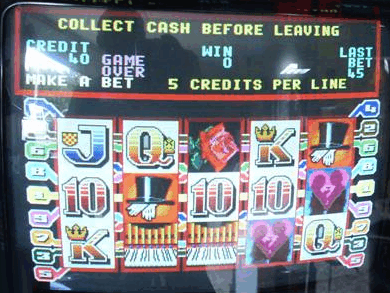## Listed and emulated in MAME !
Info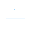(0)
Edit
Upload
### Phantom Pays © 1994 Aristocrat.

A 5-reel video slot machine with a 'Phantom of the Opera' theme.

### TECHNICAL INFORMATION

1
Aristocrat MKIV hardware.

MVP cabinet (14 button) layout :

Top row buttons :
Collect
Bet 1 Credit
Bet 2 Credits
Bet 3 Credits
Bet 5 Credits
Bet 10 Credits

Bottom row buttons :
Service
Play 1 Line / Red
Play 3 Lines
Play 5 Lines
Play 7 Lines
Play 9 Lines / Black
Gamble
Take Win

Also released on the MKV Series 1 hardware (1995).

When you get a win with a Mask substituting it displays ***PHANTOM PAY*** on the screen. The asterisks are removed on the later versions.

### SCORING

All pays are left to right, except scattered Roses which pay any.

Masks appear in reels 2, 3 and 4 and substitute for all symbols in all positions of that reel, and in one position for scattered Roses.

Paytable (Australian Local version):
Heart: 2 = 5, 3 = 100, 4 = 1000, 5 = 2000
Organ : 2 = 5, 3 = 20, 4 = 100, 5 = 1000
Candle : 2 = 5, 3 = 20, 4 = 100, 5 = 1000
Hat : 3 = 20, 4 = 50, 5 = 200
King : 3 = 10, 4 = 20, 5 = 150
Queen : 3 = 10, 4 = 20, 5 = 100
Jack : 3 = 10, 4 = 20, 5 = 100
10 : 3 = 5, 4 = 20, 5 = 100
Rose : 3 = 2, 4 = 10, 5 = 100

Paytable (New Zealand version):
Heart: 2 = 5, 3 = 50, 4 = 100, 5 = 500
Organ : 2 = 5, 3 = 20, 4 = 100, 5 = 300
Candle : 2 = 5, 3 = 20, 4 = 100, 5 = 300
Hat : 3 = 20, 4 = 50, 5 = 100
King : 3 = 10, 4 = 20, 5 = 75
Queen : 3 = 10, 4 = 20, 5 = 75
Jack : 3 = 5, 4 = 20, 5 = 50
10 : 3 = 5, 4 = 20, 5 = 50
Rose : 3 = 2, 4 = 10, 5 = 100

### TIPS AND TRICKS

If you get a win with the Mask and there is also the same symbol on a line you get the win twice for the same reel position. For instance if you spin up 3 Jacks playing 1 line and there is also the Mask symbol on reel 3, it will give you the 3x Jack win, and the 3x Jack win with the substitute.

Double Up Feature : When a win comes up, you are given the chance to bet on the credits you've just won.
If you select the right colour (Red or Black), your win is doubled. If you select the wrong item, you lose the gamble and it goes back to the main game.
You can take a win at any time, but if you win 5 times in the one gamble feature, the game ends automatically. You can't gamble more than \$10,000 however (but, would you?!).
If you win a progressive jackpot on the machine, you can't gamble the win, even if you actually won credits on the same spin the jackpot went off.

### SERIES

1. Enchanted Forest (1994)
2. Phantom Pays (1994)
3. Thunderheart (1996)
4. Mystic Eyes [Mr. Cashman] (2002)

### SOURCES

Machine's picture.
Game's ROM.